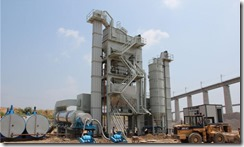﻿

储罐侧搅拌承载力检算设计

•江西益泰搅拌机械制造有限公司在江中集团的案例。搅拌设备在设计之初需要结合不同场地，搅拌物的性质，进行各项指标分析与测算，才能保证抽入生产时减少故障风险，特别是混凝土搅拌站，还要考虑周围环境是否会影响到周围，比如学校，机关等，以免造成其它单位的正常运行。
基地的地质承载能力，是否处在河道的上游会给生态带来破坏。
混凝土搅拌站最不利受力主要发生在储罐基础位置，本站设11个储罐，其中HZS180砼搅拌机配6个，HZS60砼搅拌机配5个（见图示），储罐自重按20吨考虑，基础工程拟采用桩基础。
地质资料：填土：填粉质黏土，软塑，厚5～6米，场地整平（可视作松铺未压实）；原地面：农田软塑土，厚1～1.5米，σ0=100kPa；下层： 1.5～2米范围，σ0=200kPa；次下层： 2.0～2.5米，σ0=300kPa；
一、搅拌机储罐基础设计（临近支腿间距小于0.8米） 临近支腿间距小于0.8米的搅拌机储罐基础采用9.5米φ1.5米挖孔桩(入原地面σ0=300kPa土层≥1.5米)，位于储罐四个支腿下，挖孔桩按摩擦桩设计；挖孔桩竖向承载力特征值R=3.14×1.5×(7.5×10+0.5×40+1.5×50)+3.14×0.75×0.75×300=1331KN。
单个支腿承载力F=(G水泥+G罐)/4+=(1000+200)/4=300KN,Nmax=2×F+G桩=1019.5KN＜R=1331KN。基础承载力满足要求。 二、搅拌机储罐基础设计（临近支腿间距大于0.8米）
1、搅拌机储罐基础采用8米φ1.25米挖孔桩(入原地面σ
0=200kPa
土层≥0.5米)，位于储罐四个支腿下，挖孔桩按摩擦桩设计；
挖孔桩竖向承载力特征值R=3.14×1.25×(7.5×10+0.5×40)+3.14×0.625×0.625×300=741KN。
单个支腿承载力Fmax=(G水泥+G罐)/4=(1000+200)/4=300KN Nmax=Fmax+G桩=545.3KN＜R=741KN。基础承载力满足要求。 2、搅拌机储罐基础采用9米φ1.8米挖孔桩(入原地面σ0=200kPa土层≥2.0米)，位于储罐四个支腿下，挖孔桩按摩擦桩设计；挖孔桩竖向承载力特征值R=3.14×1.8×(7.5×10+0.5×40+1×50)+3.14×0.9×0.9×300=1583KN。 单个支腿承载力F=(G 水泥 +G罐)/4=(1000+200)/4=300KN,Nmax=2 ×F+G桩=1172KN＜R=1583KN。基础承载力满足要求。 3、搅拌机储罐基础采用8.5米φ2.0米挖孔桩(入原地面σ 0=200kPa 土层≥1.5米)，位于储罐四个支腿下，挖孔桩按摩擦桩设计； 挖孔桩竖向承载力特征值R=3.14×2.0×(7.5×10+0.5×40+0.5×40)+3.14×1×1×200=1696KN。 单个支腿承载力F=(G 水泥 +G罐)/4=(1000+200)/4=300KN,Nmax=2 ×F+G桩=1267.3KN＜R=1696KN。基础承载力满足要求。 建议：1、支腿间在距混凝土面0.5内必须采用型钢进行水平连接，构成整体框架，型钢可采用双[16槽钢。 2、φ1.25米挖孔桩钢筋笼长6米，竖向采用20根φ20mm钢筋，箍筋为螺旋筋采用φ10mm钢筋，间距20cm，钢筋笼保护层8cm,主筋内侧每2米采用φ20mm钢筋设一层加强箍筋。 3、φ1.5米挖孔桩钢筋笼长9米，竖向采用30根φ20mm钢筋，箍筋为螺旋筋采用φ10mm钢筋，间距20cm，钢筋笼保护层8cm,主筋内侧每2米采用φ20mm钢筋设一层加强箍筋。

推荐 » 储罐侧搅拌承载力检算设计相关的产品微信公众服务号企业新浪微博扫我QQ了解更多